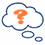# Order of OperationsPEMDAS

### Operations

"Operations" mean things like add, subtract, multiply, divide, squaring, etc. If it isn't a number it is probably an operation.

But, when you see something like ...

7 + (6 × 52 + 3)

... what part should you calculate first?

Start at the left and go to the right?
Or go from right to left?

Warning: Calculate them in the wrong order, and you can get a wrong answer !

So, long ago people agreed to follow rules when doing calculations, and they are:

## Order of Operations

Do things in Parentheses First4 × (5 + 3) = 4 × 8 = 324 × (5 + 3) = 20 + 3 = 23 (wrong)

Exponents (Powers, Roots) before Multiply, Divide, Add or Subtract5 × 22 = 5 × 4 = 205 × 22 = 102 = 100 (wrong)

Multiply or Divide before you Add or Subtract2 + 5 × 3 = 2 + 15 = 172 + 5 × 3 = 7 × 3 = 21 (wrong)

Otherwise just go left to right30 ÷ 5 × 3 = 6 × 3 = 1830 ÷ 5 × 3 = 30 ÷ 15 = 2 (wrong)

## How Do I Remember It All ... ? PEMDAS !

 P Parentheses first E Exponents (ie Powers and Square Roots, etc.) MD Multiplication and Division (left-to-right) AS Addition and Subtraction (left-to-right)

Divide and Multiply rank equally (and go left to right).

Add and Subtract rank equally (and go left to right)

So do it this way:After you have done "P" and "E", just go from left to right doing any "M" or "D" as you find them.

Then go from left to right doing any "A" or "S" as you find them.You can remember by saying "Please Excuse My Dear Aunt Sally". Or ... Pudgy Elves May Demand A Snack Popcorn Every Monday Donuts Always Sunday Please Eat Mom's Delicious Apple Strudels People Everywhere Made Decisions About Sums

Note: in the UK they say BODMAS (Brackets,Orders,Divide,Multiply,Add,Subtract), and in Canada they say BEDMAS (Brackets,Exponents,Divide,Multiply,Add,Subtract). It all means the same thing! It doesn't matter how you remember it, just so long as you get it right.

## Examples

### Example: How do you work out 3 + 6 × 2 ?

First 6 × 2 = 12, then 3 + 12 = 15

### Example: How do you work out (3 + 6) × 2 ?

Parentheses first:

First (3 + 6) = 9, then 9 × 2 = 18

### Example: How do you work out 12 / 6 × 3 / 2 ?

Multiplication and Division rank equally, so just go left to right:

First 12 / 6 = 2, then 2 × 3 = 6, then 6 / 2 = 3

A practical example:### Example: Sam threw a ball straight up at 20 meters per second, how far did it go in 2 seconds?

Sam uses this special formula that includes the effects of gravity:

height = velocity × time − (1/2) × 9.8 × time2

Sam puts in the velocity of 20 meters per second and time of 2 seconds:

height = 20 × 2 − (1/2) × 9.8 × 22

Now for the calculations!

Parentheses first:20 × 2 − 0.5 × 9.8 × 22
Then Exponents (22=4):20 × 2 − 0.5 × 9.8 × 4
Then the Multiplies:4019.6
Subtract and DONE !20.4

The ball reaches 20.4 meters after 2 seconds

## Exponents of Exponents ...

432

Exponents are special: they go top-down (do the exponent at the top first). So we calculate this way:

So 432 = 4(32), not (43)2

And finally, what about the example from the beginning?